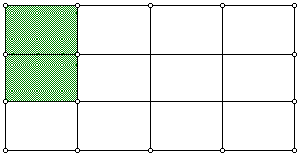# Let’s Operate on Fractions!

Using an area model for fractions*, explain how the image below shows a particular operation is being performed on the given fractions, 2/3 and 1/4.How does the area model help explain why it makes sense to multiply the denominators and why it makes sense to multiply the numerators?

*Consider using Fraction Bars, Geometers’ SketchPad, or manipulatives.